Open in App
Not now

# Simple Harmonic Motion

• Difficulty Level : Basic
• Last Updated : 14 Jul, 2021

The study of oscillatory motion is fundamental to physics; its concepts are required to comprehend many physical phenomena. Vibrating strings produce pleasing sounds in musical instruments such as the sitar, guitar, and violin. Membranes in drums and diaphragms in telephone and speaker systems vibrate about their mean positions. Let’s briefly discuss oscillatory motion and simple harmonic motion.

### What is an Oscillatory Motion?

The to and fro motion of an object from its mean position is defined as oscillatory motion. The ideal condition is for the object to remain in oscillatory motion in the absence of friction indefinitely, but this is not possible in the real world, and the object must settle into equilibrium. The term vibration, which is found in a swinging pendulum, is used to describe mechanical oscillation. Similarly, the human heartbeat is an example of oscillation in dynamic systems.

• An oscillatory motion is defined as a particle moving to and fro about a mean position. An oscillatory motion is defined as a particle moving on either side of equilibrium (or) mean position.
• It is a type of periodic motion with two extreme points. For instance, consider the Oscillation of a Simple Pendulum and the Spring-Mass System.
• The object will continue to move between two extreme points around a fixed point, which is known as the mean position (or) equilibrium position along any path. (Note that the path is not a constraint.)
• There will be a restoring force directed at the equilibrium (or mean) position.
• The net force on the particle in an oscillatory motion is zero at the mean position.
• The mean position is an equilibrium position that is stable.

Some Examples of Oscillatory Motion are:

1. Simple pendulum oscillation.
2. The vibrating strings of musical instruments are a mechanical example of oscillatory motion.
3. Spring movement.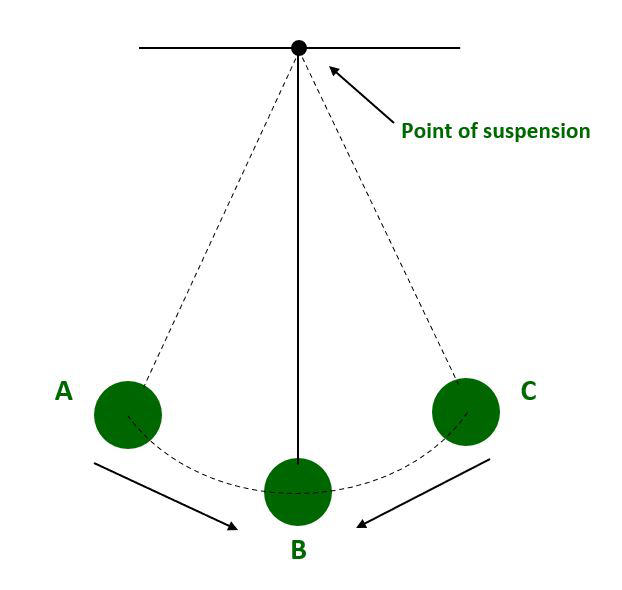Oscillation of simple pendulum.

### What is a Periodic Motion?

In general, we describe the motion of bodies in terms of how they move. The motion of an object is said to be periodic if it moves in such a way that it repeats its path at regular intervals of time.

• After an equal interval of time, a motion repeats itself. Consider uniform circular motion.
• There is no such thing as an equilibrium position.
• There is no such thing as a restoring force.
• There is no such thing as a stable equilibrium position.

Some Examples of Periodic Motion are:

1. The movement of a clock’s hands. The period of motion of an hour’s hand is 12 hours, that of a minute’s hand is one hour, and that of a clock’s second hand is one minute.
2. A simple pendulum is said to be periodic when it is pulled from its rest position to one side and released, causing to and fro motion (oscillatory motion).
3. The movement of the moon around the Earth.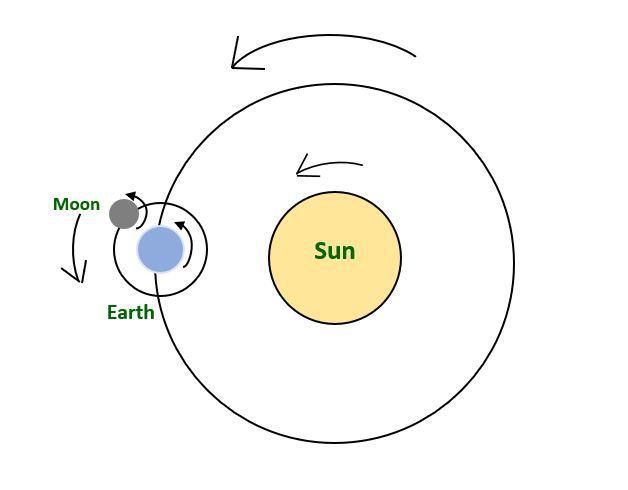Periodic Motion of Earth around the sun

### Simple Harmonic Motion (SHM)

Simple harmonic motion is an oscillatory motion in which the particle’s acceleration at any position is directly proportional to its displacement from the mean position. It is an example of oscillatory motion.

Simple Harmonic Motions (SHM) are all oscillatory and periodic, but not all oscillatory motions are SHM. Oscillatory motion is also referred to as the harmonic motion of all oscillatory motions, the most important of which is simple harmonic motion (SHM).

• It is a type of oscillation with a straight line connecting the two extreme points (the path of SHM is a constraint).
• The object’s path must be a straight line.
• There will be a restoring force directed at the equilibrium (or mean) position.
• In Simple harmonic motion, the mean position is a stable equilibrium.

(1) Linear Simple Harmonic Motion:

When a particle moves back and forth along a straight line around a fixed point (called the equilibrium position), this is referred to as Linear Simple Harmonic Motion. As an example, consider the spring-mass system.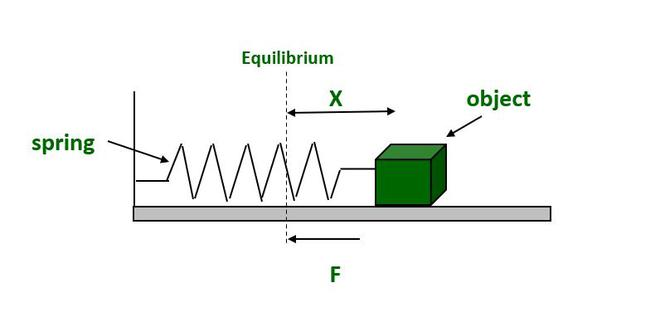Conditions for Linear Simple Harmonic Motion

The restoring force or acceleration acting on the particle must always be proportional to the particle’s displacement and directed towards the equilibrium position.

F ∝ – X

or

a ∝ -x

where F is the Restoring force, X is the displacement of particle from equilibrium position and a is the acceleration.

(2) Angular Simple Harmonic Motion:

An angular simple harmonic motion occurs when a system oscillates angularly long with respect to a fixed axis. The displacement of the particle in angular simple harmonic motion is measured in terms of angular displacement. The torsional pendulum is an example.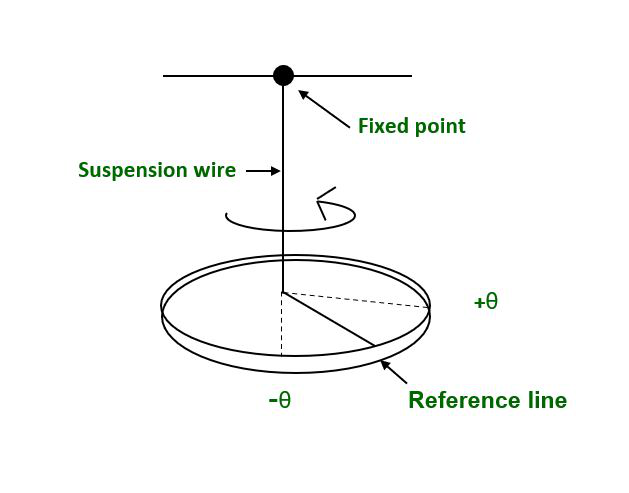Torsional pendulum

Conditions for Angular Simple Harmonic Motion:

The restoring torque (or) angular acceleration acting on the particle should always be proportional to the particle’s angular displacement and oriented towards the equilibrium position.

T ∝ -θ

or

α ∝ -θ

where T is Torque, θ is the Angular displacement and α is the Angular acceleration.

### Terminology related to Simple Harmonic Motion

• Amplitude: The amplitude of a particle is its maximum displacement from its equilibrium or mean position, and its direction is always away from the mean or equilibrium position. The meter is its SI unit.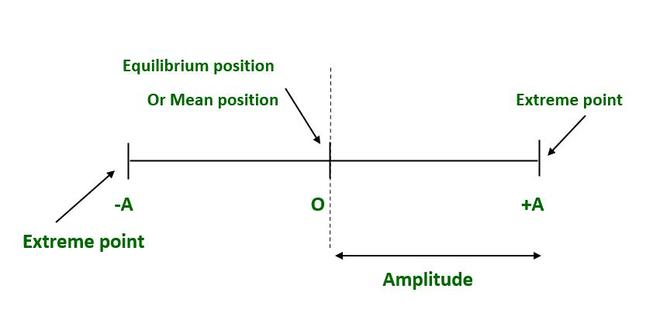• Time Period: The period of a particle is the amount of time it takes to complete one oscillation. As a result, the period of SHM is the shortest time before the motion repeats itself. As a result, the motion will repeat after nT, where n is an integer.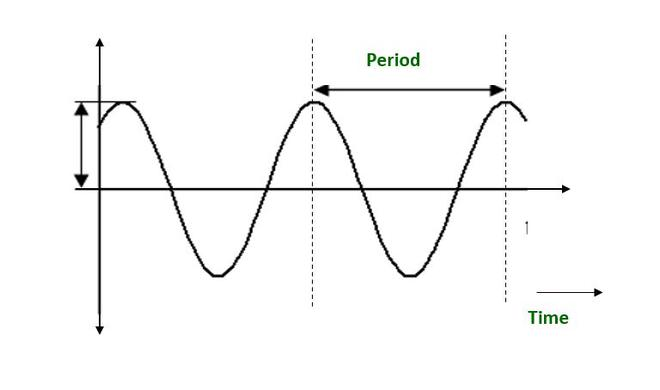T = 2π/ω

where ω is the Angular frequency and T is the Time period.

• Frequency: The frequency of SHM is the number of oscillations performed by a particle per unit of time. Hertz, or r.p.s. (rotations per second), is the SI unit of frequency.

Frequency, f = 1/ T and

Angular frequency, ω = 2πf = 2π/T

• Phase: The magnitude and direction of particle displacement represent the phase of SHM, which is its state of oscillation.

The expression for a particle’s position as a function of time.

x = A sin (ωt + Φ)

where (ωt + Φ) is the particles phase.

• Phase Difference: The phase difference is defined as the difference between the total phase angles of two particles moving in simple harmonic motion with respect to the mean position. When two vibrating particles are in the same phase, their phase difference is an even multiple of π. If the phase difference between two vibrating particles is an odd multiple of π, they are said to be in the opposite phase.

Phase Difference is represented as ΔΦ.

ΔΦ = nπ                                                                                           [for same phase]

ΔΦ = (2n + 1) π                                                                               [for odd phase]

where n = 0, 1, 2, 3, . . . . .

### Equations for Simple Harmonic Motion

Lets consider a particle of mass (m) doing Simple Harmonic Motion along a path A’OA the mean position is O. Let the speed of the particle be V0 when it is at position P (at some distance from point O)

At the time, t = 0 the particle at P (moving towards point A)

At the time, t = t the particle is at Q (at a distance X from point O) at this point if velocity is V then: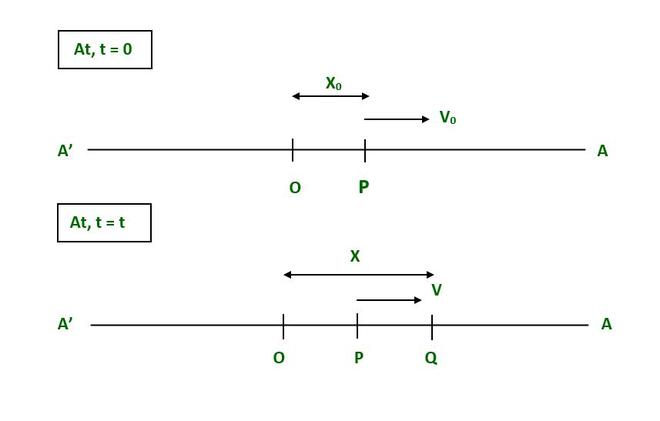The force F acting on a particle at point p is given as,

F = -K X                                                                                                 where, K = positive constant

We know that,

F = m a                                                                                                  where, a = Acceleration at Q

m a = -K X

a = -(K/m) X                                                                                         [putting ω2 = K/m]

a = -ω2 X                                                                                             [Since, a = d2X/d2t]

d2X/d2t  =  -ω2 X

Hence,

d2X/d2t + ω2 X = 0

which is the differential equation for linear simple harmonic motion.

Solutions of Differential Equations of SHM:

The solutions to the differential equation for simple harmonic motion are as follows:

• This solution when the particle is in its mean position at point (O): x = Asinωt.
• When the particle is at the position p (not at mean position): x = Asinϕ.
• When the particle is at position Q (any time t): x = Asin(ωt+ϕ).

### Sample Problems

Problem 1: Why is Harmonic Motion Periodic?

Solution:

The sine wave can represent a harmonic motion. When a spring is stretched from its mean position, it oscillates to and fro about the mean position under the influence of a restoring force that is always directed towards the mean position and whose magnitude at any instant is proportional to the body’s displacement from the mean position at that instant. When there is no friction, the motion tends to be periodic. The harmonic motion is periodic in this case.

Problem 2: What are Periodic and Non-Periodic Changes?

Solution:

Periodic changes are those that occur at regular intervals of time, such as the occurrence of day and night, or the change of periods in your school. Non-periodic changes are those that do not occur on a regular basis, such as the freezing of ice to water.

Problem 3: What is the period of the earth’s revolution around the sun and around its polar axis? what is the motion earth performs explain?

Solution:

The earth’s revolution around the sun takes one year, and its revolution around its polar axis takes one day. The motion of earth is periodic because after some interval of time it repeats its path.

Problem 4: Is it possible for a motion to be oscillatory but not simple harmonic?

Solution:

When a ball is dropped from a height onto a perfectly elastic surface, the motion of the ball is oscillatory but not simple harmonic because the restoring force, F = mg = constant.

Problem 5: What is the frequency in SHM. How time periods and frequency are related?

Solution:

The frequency of SHM is the number of oscillations performed by a particle per unit of time. Hertz, or r.p.s. (rotations per second), is the SI unit of frequency. Frequency and time period are related as:

Frequency, (f) = 1/ Time period (T)

Problem 6: What is the angular frequency in simple harmonic motion?

Solution:

Angular frequency (ω), also known as radial or circular frequency, is a unit of time for measuring angular displacement. As a result, its units are degrees (or radians) per second.

The angular frequency(ω) is given by the expression,

ω = 2π/T.

Problem 7: A spring with a spring constant of 1200 N m–1 is mounted on a horizontal table. A 3 kg mass is attached to the free end of the spring. The mass is then pulled sideways to a distance of 2.0 m before being released. Determine the following:

(i) The frequency of oscillations,

(ii) Maximum acceleration of the mass, and

(iii) The maximum speed of the mass.

Solution: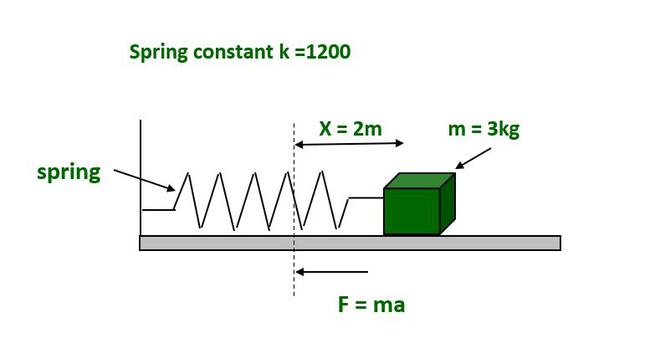Given that,

The spring constant, k = 1200 N/m.

The mass of the object, m = 3 kg.

The displacement, x = 2 m.

(i) The frequency of oscillation:

We know that frequency (f) = 1/Time period (T)                                                          [ T = 2π/ω and ω = √k/m]

Therefore,

f = (1/2π)√k/m

= (1/2 × 3,14) √1200/3 = 3.18 Hz.

(ii) Maximum acceleration of the mass:

The Maximum acceleration (a) = ω2x

where, ω = Angular frequency = √k/m

Therefore, a = x(k/m)

a = 2 × (1200/3) = 800 m/s2.

(iii) The maximum speed of the mass:

The maximum speed (V) = ωx

Put, ω = √k/m.

Therefore, V = x(√k/m)

V = 2 × (√1200/3) = 40 m/s.

My Personal Notes arrow_drop_up